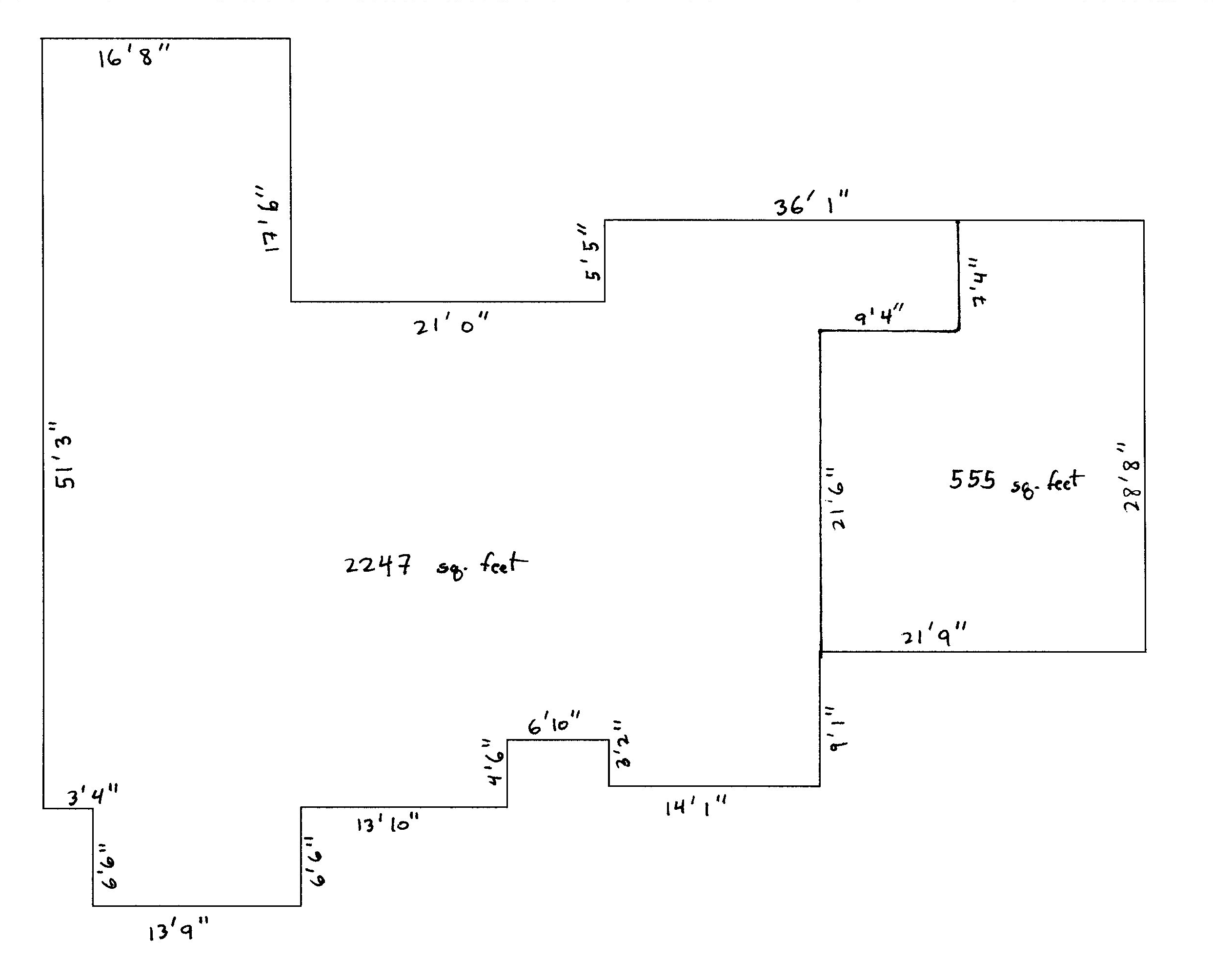# Details

Suppose we want to find the area of a region $$X$$, where $$X \subset Y = [a,b] \times [c,d]$$. Let $$|X|$$ and $$|Y|$$ denotes the areas of $$X$$ and $$Y$$, respectively. Let $$Y_1$$ and $$Y_2$$ be independent and uniformly distributed random variables on the intervals $$[a,b]$$ and $$[c,d]$$, respectively. Then, $\text{Pr}( (Y_1,Y_2) \in X) = \frac{|X|}{|Y|}$ Isolating $$|X|$$, we have: \begin{align*} |X| & = |Y| \cdot \text{Pr}( (Y_1,Y_2) \in X) \\ & = (b-a) (d-c) \cdot \text{Pr}( (Y_1,Y_2) \in X) \\ & = (b-a) (d-c) \cdot \text{E}( \text{I}\{(Y_1,Y_2) \in X\}). \\ & = \text{E}( (b-a) (d-c) \cdot \text{I}\{(Y_1,Y_2) \in X\}). \\ \end{align*} Now that $$|X|$$ is written as an expectation, we can use Monte Carlo integration to estimate it.

Specifically, the “hit-and-miss” method is a follows: Uniformly sample B points in $$Y$$. The Monte Carlo estimate of $$|X|$$ is the proportion of points in $$X$$ times $$|Y|$$ (i.e., $$(b-a) \cdot (d-c)$$).

# Example 1: Estimate $$\pi$$

Suppose one wishes to find the value of $$\pi$$ using the hit-and-miss method. Consider the unit cirle, that is, a circle having radius 1 centered at the origin. Theoretically, the area of the circle is $$\pi$$. Let’s us the hit-and-miss algorithm to estimate $$\pi$$. The square $$Y = [-1,1] \times [-1,1]$$ has area $$2 \times 2 = 4$$ and the unit circle is a subset of it.

B <- 1000000
x <- 4 * ( runif(B)^2 + runif(B)^2 <= 1 )
pi.est <- mean(x)
pi.est
##  3.142788
pi.ci <- pi.est + c(-1,1)*qnorm(0.975)*sd(x)/sqrt(B)
pi.ci
##  3.139571 3.146005
pi   # Actual value
##  3.141593

# Example 2: Area of my Texas home# Extensions

Could this be applied to $$\mathcal{R}^3$$ to get volumes? What problems might there be as we increase the number of dimensions?